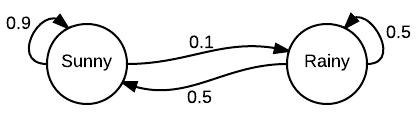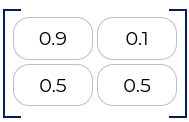#### What are Markov Chains, why are they useful, and how to implement them

Imagine the following scenario: you want to know whether the weather tomorrow is going to be sunny or rainy. Now, you might have a natural intuition based on your experience and historical observation of the weather. If for the past 1 week the weather has been sunny, then you have a 90% certainty that tomorrow will also be sunny. But if for the previous week or so the weather has been rainy, then the probability of tomorrow being sunny does not look too good: only at 50% chance. This scenario can be described as a Markov Chain process.Photo by Shaojie on Unsplash

### What is Markov Chain?

But what is a Markov Chain, formally? Markov Chain is a mathematical system that describes a collection of transitions from one state to the other according to certain stochastic or probabilistic rules.

Take for example our earlier scenario for predicting the next day’s weather. If today’s weather is sunny, then based on our (reliable) experience, the probability for the weather tomorrow transitioning to rainy is 10% and sunny 90%. On the other hand, if at present the weather is rainy, then the probability for tomorrow remaining rainy is 50% and sunny 50%.

These changes (or the lack thereof) between different states are called transitions while the variable of interest (ie. rainy or sunny) are called states.Weather transitions that follow Markov rule

For these transitions, however, to be qualified as Markov Chain, they must satisfy the Markov Property. The property states that the probability of transition is entirely dependent only on the current state, and not on the preceding set of sequences. This characteristic allows Markov Chain to be memory-less.

### Why Markov Chain?

Markov Chain has many applications in the real-world processes, from game theory, physics, economics, signal processing, to information theory.

Furthermore, this seemingly simple process serves as the basis for many more complex stochastic simulation methods such as Markov Chain Monte Carlo (MCMC). Markov Chain is also a precursor for many of the modern data science techniques, such as the building block for Bayesian statistics.

In all, Markov Chain will serve as a good starting point for you to understand more advanced statistical modelling techniques in data science.

### More About Markov Chain: Mathematical Definition

The Markov Chain model represents the probabilities for state transitions as a transition matrix. If the system has N possible states (eg. N=2 for our weather prediction case), then the transition matrix will have a N x N shaped transition matrix. Subsequently, the individual entry for the matrix, N(i, j), will indicate the probability of transition between state i and state j.

For our weather prediction case, the transition matrix, T can be illustrated as:Transition Matrix, T, for the weather prediction problem
What will happen if you want to determine the probability over multiple steps, say for the weather to rain over the next M days? You can simply raise the transition probability to the power of M.

### Programming Markov Chain

Let’s try to code the above example in Python.

1. Import the necessary libraries
`import numpy as npimport random as rm`

2. Define the states and their probabilities (NOTE: ensure that the total probability in each row sums up to 1)

`states = ["sunny", rainy"]transitions = [["SS", "SR"],["RS", "RR"]]`
`T = [[0.9, 0.1],[0.5, 0.5]]`

3. Lets write the (rather tedious) Markov Chain function to predict the weather for the next n number of days! (NOTE: you should probably look for better Python library that abstracts the implementation of Markov Chain).

`def weather_forecast(n_days, weather_today="sunny"):    weather_list = [weather_today]    n = 0    prob = 1.0`
`    while n != n_days:        if weather_today == "sunny":            change = np.random.choice(transitions, p=T)            if change == "SS":                prob = prob * T                weather_list.append(states)            else:                prob = prob * T                weather_list.append(states)`
`        else:            weather_change=np.random.choice(transition,p=T)            if change == "RS":                prob = prob * T                weather_list.append(states)            else:                prob = prob * T                weather_list.append(states)`
`        n = n + 1`
`        return weather_list`

4. Run the program, say, for the next 5 days.

`future_weathers = weather_forecast(n_days = 5)`

### Conclusion

Now that you are familiar with how a Markov Chain works, you can deep dive into more complex stochastic modelling techniques, such as the Hidden Markov Process or MCMC. Stay tuned for more of these follow-up contents!Introduction to Markov Chain Programming was originally published in Towards Data Science on Medium, where people are continuing the conversation by highlighting and responding to this story.